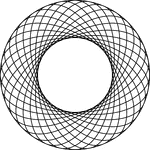### 16 Rotated Concentric Ellipses

Illustration of 16 concentric congruent ellipses that are rotated about the center at equal intervals…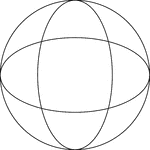### 2 Rotated Concentric Ellipses

Illustration of 2 concentric congruent ellipses that are rotated about the center at 90°. The ellipses…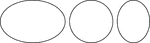### 2 Ellipses With Equal Vertical Axes

An illustration of 2 ellipses that have the equal vertical axes, but different horizontal axes. The…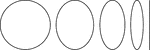### 2 Ellipses With Equal Vertical Axes

An illustration of 2 ellipses that have the equal vertical axes, but different horizontal axes. The…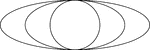### 3 Concentric Ellipses

An illustration of 3 concentric ellipses that are tangent at the end points of the vertical axes. The…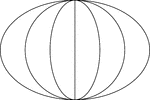### 3 Concentric Ellipses

An illustration of 3 concentric ellipses that are tangent at the end points of the vertical axes, which…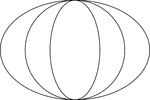### 3 Concentric Ellipses

An illustration of 3 concentric ellipses that are tangent at the end points of the vertical axes. The…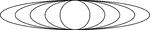### 4 Concentric Ellipses

An illustration of 4 concentric ellipses that are tangent at the end points of the vertical axes. The…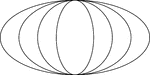### 4 Concentric Ellipses

An illustration of 4 concentric ellipses that are tangent at the end points of the vertical axes. The…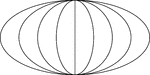### 4 Concentric Ellipses

An illustration of 4 concentric ellipses that are tangent at the end points of the vertical axes, which…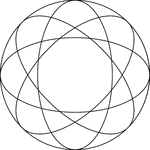### 4 Rotated Concentric Ellipses

Illustration of 4 concentric congruent ellipses that are rotated about the center at equal intervals…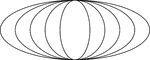### 5 Concentric Ellipses

An illustration of 5 concentric ellipses that are tangent at the end points of the vertical axes. The…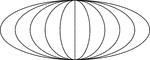### 5 Concentric Ellipses

An illustration of 5 concentric ellipses that are tangent at the end points of the vertical axes, which…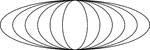### 6 Concentric Ellipses

An illustration of 6 concentric ellipses that are tangent at the end points of the vertical axes. The…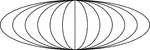### 6 Concentric Ellipses

An illustration of 6 concentric ellipses that are tangent at the end points of the vertical axes, which…### 8 Rotated Concentric Ellipses

Illustration of 8 concentric congruent ellipses that are rotated about the center at equal intervals…